Geometry

Area arbitrary quadrilateral, formulas and a calculator for calculating area online and a summary table with area formulas of the quadrilateral. To calculate area of an arbitrary quadrilateral, various formulas are used, depending on the known source data. Below are formulas and a calculator that will help you calculate area of an arbitrary quadrilateral or check already completed calculations.

At the end of the article there are links for calculating particular cases of quadrilaterals: square, trapezoid, parallelogram, rectangle, rhombus.

Table with area quadrilateral formulas (at the end of the page)

1

## Area quadrilateral along the diagonals and the angle between them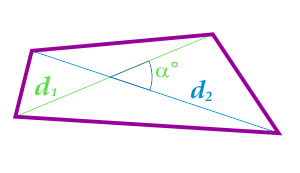... preparation ...
d1 - diagonal
d2 - diagonal
α° - Angle between diagonals

2

## Area quadrilateral through the sides and the angles between these sides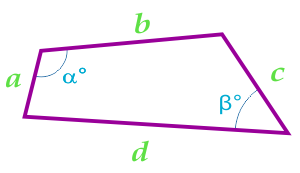When calculating area quadrilateral using this formula, you must first calculate the perimeter of the quadrilateral by the formula:

... preparation ...

a - side
b - side
c - side
d - side
α° - angle between parties
β° - angle between parties

3

## Area quadrilateral inscribed in a circle, calculated according to the Brahmagupta Formula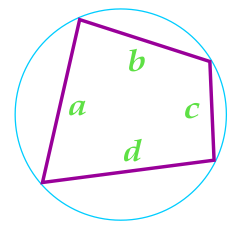This formula is valid only for quadrilaterals around which a circle can be described.

When calculating area quadrilateral using this formula, you must first calculate the perimeter of the quadrilateral by the formula:

... preparation ...

a - side
b - side
c - side
d - side

4

## Area quadrilateral into which the circle can be entered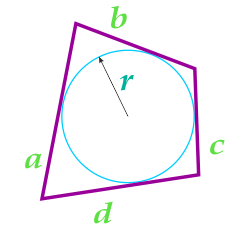This formula is valid only for quadrilaterals into which you can enter a circle. The inscribed circle must have points of contact with all four sides of the quadrilateral.

When calculating area quadrilateral using this formula, you must first calculate the perimeter of the quadrilateral by the formula:

... preparation ...
a - side
b - side
c - side
d - side
r - radius of inscribed circle

5

## Area quadrilateral into which the circle defined by the sides and the angles between them can be entered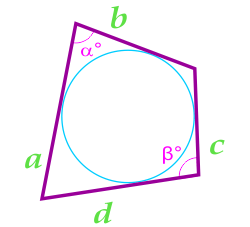This formula is valid only for quadrilaterals into which you can enter a circle. The inscribed circle must have points of contact with all four sides of the quadrilateral.

... preparation ...

a - side
b - side
c - side
d - side
α° - angle between parties
β° - angle between parties

Note:

If the angle is specified in radians in the source data, then for conversion to degrees you can use the formula: 1 radian × (180/π)° = 57,296°

## Table with area quadrilateral formulas

 initial data(active link to go to the calculator) sketch formula 1 diagonal and angle between them 2 sides and angles between these sides where  , 3 the parties where 4 sides and radius of the inscribed circle where 5 sides and angles between them

## Area of special cases of quadrilaterals

To calculate special cases of quadrilaterals, you can use the formulas and calculators given in other articles of the site: